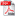# VECTORIAL PROBABILISTIC MODEL FOR THE UNBALANCE FACTOR OF A THREE-PHASE ELECTRICAL NETWORK

Autor/autori: Lambert Pierrat (Franta)

Abstract: In a three-phase power electrical network, the voltage asymmetry can be split into two different components. The first component results from the network structure and possesses almost constant value caused by asymmetry of circuit elements (e.g., lines, transformers, etc.). The second component is a quasi-stationary fluctuation process because it comes from temporary asymmetry of electric loads, random switching on/off of single phase loads, transient response of three phase loads. For the previous reasons, a probabilistic vectorial model is proposed to take into account the random properties of voltage asymmetry. The probability distribution of its magnitude is determined by the two previous components, each one being characterized by its specific parameter. Finally, a new simple criterion characterizing asymmetry is proposed, useful for electrical power quality study.

Keywords: electrical power network, 3-phase unbalance, structural asymmetry, functional asymmetry, fluctuation process, unbalance factor, exceeding probabilityDOWNLOAD PDF